Courses

Test: Introduction To Arithmetic Progression

20 Questions MCQ Test Mathematics (Maths) Class 10 | Test: Introduction To Arithmetic Progression

Description
Attempt Test: Introduction To Arithmetic Progression | 20 questions in 20 minutes | Mock test for Class 10 preparation | Free important questions MCQ to study Mathematics (Maths) Class 10 for Class 10 Exam | Download free PDF with solutions
QUESTION: 1

Solution:
QUESTION: 2

Solution:
QUESTION: 3

If p, q, r, s, t are the terms of an A.P. with common difference -1 the relation between p and t is:​

Solution:

p, q, r, s, t are the terms of AP. So a=p , d= -1
To find a relation between t and p we use
l=a+(n-1)d, where l=t and n=5
t=p+(5-1)(-1)=p+4(-1)=p-4
This is because the difference is negative so it will be smaller than p.

QUESTION: 4

The weights of 11 students selected for a team are noted in ascending order and are in A. P. The lowest value is 45 Kg, and the middle value is 55 Kg. What is the difference between the two values placed consecutively ?

Solution:
QUESTION: 5

Is the sequence, whose general term is 5n2 + 2n + 3 an AP?​

Solution:

We have the sequence 5n2+2n+3
It will be in AP if it satisfies an-an-1=d where d is a constant.
Here, an=5n2+2n+3
And, an-1=5(n-1)2+2(n-1)+3=5(n2+1-2n)+2n-2+3
=5n2+5-10n+2n+1=5n2-8n+6
an-an-1=5n2+2n+3-(5n2-8n+6)
=5n2+2n+3-5n2+8n-6
=10n-3, which depends on a variable , so its not constant.

QUESTION: 6

Find the next two terms of the A.P.:- -10, -6,-2…

Solution:

We have A.P.: -10, -6,-2…
So a= -10, d=-6+10=-2+6=4
l=a+(n-1)d
For fourth term,
= -10+(3)*4=-10+12=2
For 5th term
= -10+4*4=6

QUESTION: 7

If a + 1, 2a + 1, 4a – 1 are in A.P., then value of ‘a’ is:

Solution: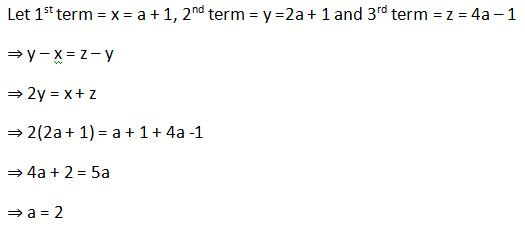QUESTION: 8

Give that an A.P. has term as 5 and common differences as 2. What is the A.p.?​

Solution:

A¹=5 d=2
a²=a¹+d =5+2=7
a³=a²+d =7+2 =9
a⁴=a³+d=9+2=11
A5 =a⁴+d=11+2=13
Therefore 5,7,9,11,13 is an AP.

QUESTION: 9

The 5th term of an A.P. is 18, the common difference is 2. What is the first term ?

Solution:

5th term of AP is 18 and common difference is 2
We have, l = a + (n - 1)d
Where l = 18,a = ?,n = 5,d = 2
Substituting the values,
18 = a+8
a = 10

QUESTION: 10

Ramesh’s salary in February 2008 is Rs. 10,000. If he’s promised an increase of Rs. 1000 every year, what would be his salary in Feb 2011?​

Solution:

So this is an AP with a=10,000 , d= 1000
So after 4 years
l=a+(n-1)d=10000+(4 -1)1000=10000+3000=13000

QUESTION: 11

Given an A.P. few of whose terms are x, y, 2, 4, 6, 8,………. What must be the values of x and y?​

Solution:

Arithmetic progression is a sequence of numbers such that the difference of any two successive members is a constant,d
AP is x, y, 2, 4, 6, 8,…
So we have 4-2=2,6-4=2 so we have d=2
So y=2-2=0
And x=0-2=-2

QUESTION: 12

If p – 1, p + 3, 3p – 1 are in A.P., then p is equal to:

Solution:

We have given that
p-1,p+3 , 3p-1 are in A.P

we have to find p= ?

solution :-
we know that :
if a,b,c are in AP
then 2b = a + c

here
=> 2(p+3) = { (p-1) + ( 3p -1) }

=> 2p +6 = 4p -2

=> 2p = 8

=> p = 4

QUESTION: 13

Which of the following is an A.P with -10 as first term and 2 as the common difference?​

Solution:
QUESTION: 14

Amit starts his exercise regime with 25 push ups on Monday. He plans to increase 5 push ups every following Monday. How many push ups will he be doing on the 3rd Monday since he started?​

Solution:

On 1st Monday he did 25
on 2nd next he did 25+5=30
on 3rd Monday he did 30+5=35

QUESTION: 15

For what values of k can the following numbers form an A.P:2k-1,k+2,2k​

Solution:

If  2k-1,k+2,2k are in AP, the difference of two consecutive terms will be same.
Therefore,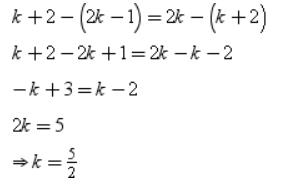QUESTION: 16

From an A.P. 5 consecutive terms are required to be written. The central term out of the five terms is given as 5 and it’s informed that the common difference of the A.P. is 4. Write the 5 terms in the correct sequence.​

Solution:

AP is:  a,a+d,a+2d,a+3d,a+4d
Middle term is a+2d=5
d=4
a+2d=5
a+2*4=5
a=5-8= -3
a+d= -3+4=1
a+3d= -3+3*4=-3+12=9
a+4d= -3+4*4= -3+16=13
So the terms are : -3,1,5,9,13

QUESTION: 17

If 4/5, a, 2 are three consecutive terms of an A.P., then the value of a is:

Solution:
QUESTION: 18

If a - b, 0 and a + b are consecutive terms of an AP then

Solution:
QUESTION: 19

The next term of the A.P. ​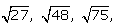… is:

Solution:

AP is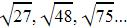So a =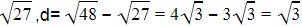So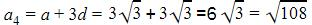QUESTION: 20

The angles of a triangle in A.P. the smallest being half of the greatest. So what are the angles ?​

Solution:

Arithmetic progression is a sequence of numbers such that the difference of any two successive members is a constant,d.
So let the angles be a-d , a and a+d
We are given smallest angle is half of the largest
So, a-d=1/2(a+d)
2(a-d)=a+d
2a-2d=a+d
a=3d
Using angle sum property,
(a-d)+a+(a+d)=180
3a=180
a=60
a=3d
3d=60
d=20
a-d=60-20=40
a=60
a+d=60+20=80
So , the angles are 40,60,80Use Code STAYHOME200 and get INR 200 additional OFF Use Coupon Code

Track your progress, build streaks, highlight & save important lessons and more!

Similar ContentRelated tests